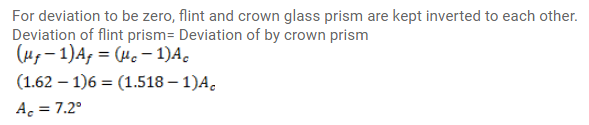# A flint glass prism and a crown glass prism are to be combinedQuestion:

A flint glass prism and a crown glass prism are to be combined in such a way that the deviation of the mean ray is zero. The refractive index of flint and crown glasses for the mean ray are $1.620$ and $1.518$ respectively. If the refracting angle of the flint prism is $6.0^{\circ}$, what would be the refracting angle of the crown?

Solution: